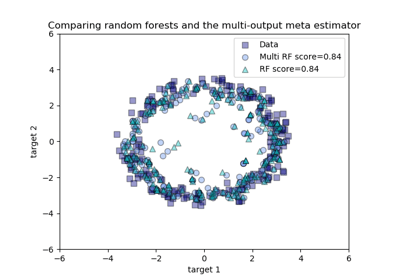/scikit-learn

# sklearn.multioutput.MultiOutputRegressor

`class sklearn.multioutput.MultiOutputRegressor(estimator, n_jobs=None)` [source]

Multi target regression

This strategy consists of fitting one regressor per target. This is a simple strategy for extending regressors that do not natively support multi-target regression.

Parameters: `estimator : estimator object` An estimator object implementing `fit` and `predict`. `n_jobs : int or None, optional (default=None)` The number of jobs to run in parallel for `fit`. `None` means 1 unless in a `joblib.parallel_backend` context. `-1` means using all processors. See Glossary for more details. When individual estimators are fast to train or predict using `n_jobs>1` can result in slower performance due to the overhead of spawning processes.

#### Methods

 `fit`(X, y[, sample_weight]) Fit the model to data. `get_params`([deep]) Get parameters for this estimator. `partial_fit`(X, y[, sample_weight]) Incrementally fit the model to data. `predict`(X) Predict multi-output variable using a model trained for each target variable. `score`(X, y[, sample_weight]) Returns the coefficient of determination R^2 of the prediction. `set_params`(**params) Set the parameters of this estimator.
`__init__(estimator, n_jobs=None)` [source]
`fit(X, y, sample_weight=None)` [source]

Fit the model to data. Fit a separate model for each output variable.

Parameters: `X : (sparse) array-like, shape (n_samples, n_features)` Data. `y : (sparse) array-like, shape (n_samples, n_outputs)` Multi-output targets. An indicator matrix turns on multilabel estimation. `sample_weight : array-like, shape = (n_samples) or None` Sample weights. If None, then samples are equally weighted. Only supported if the underlying regressor supports sample weights. `self : object`
`get_params(deep=True)` [source]

Get parameters for this estimator.

Parameters: `deep : boolean, optional` If True, will return the parameters for this estimator and contained subobjects that are estimators. `params : mapping of string to any` Parameter names mapped to their values.
`partial_fit(X, y, sample_weight=None)` [source]

Incrementally fit the model to data. Fit a separate model for each output variable.

Parameters: `X : (sparse) array-like, shape (n_samples, n_features)` Data. `y : (sparse) array-like, shape (n_samples, n_outputs)` Multi-output targets. `sample_weight : array-like, shape = (n_samples) or None` Sample weights. If None, then samples are equally weighted. Only supported if the underlying regressor supports sample weights. `self : object`
`predict(X)` [source]
Predict multi-output variable using a model
trained for each target variable.
Parameters: `X : (sparse) array-like, shape (n_samples, n_features)` Data. `y : (sparse) array-like, shape (n_samples, n_outputs)` Multi-output targets predicted across multiple predictors. Note: Separate models are generated for each predictor.
`score(X, y, sample_weight=None)` [source]

Returns the coefficient of determination R^2 of the prediction.

The coefficient R^2 is defined as (1 - u/v), where u is the residual sum of squares ((y_true - y_pred) ** 2).sum() and v is the regression sum of squares ((y_true - y_true.mean()) ** 2).sum(). Best possible score is 1.0 and it can be negative (because the model can be arbitrarily worse). A constant model that always predicts the expected value of y, disregarding the input features, would get a R^2 score of 0.0.

Parameters: `X : array-like, shape (n_samples, n_features)` Test samples. `y : array-like, shape (n_samples) or (n_samples, n_outputs)` True values for X. `sample_weight : array-like, shape [n_samples], optional` Sample weights. `score : float` R^2 of self.predict(X) wrt. y.

#### Notes

R^2 is calculated by weighting all the targets equally using `multioutput=’uniform_average’`.

`set_params(**params)` [source]

Set the parameters of this estimator.

The method works on simple estimators as well as on nested objects (such as pipelines). The latter have parameters of the form `<component>__<parameter>` so that it’s possible to update each component of a nested object.

Returns: self

## Examples using `sklearn.multioutput.MultiOutputRegressor`Comparing random forests and the multi-output meta estimator